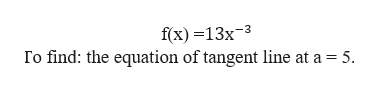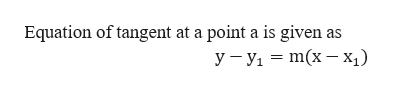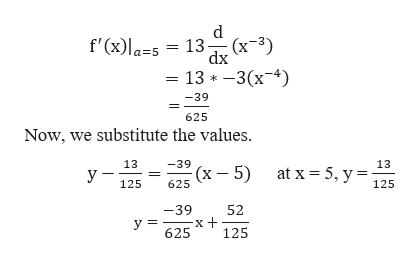Compute f and find anequation of the tangent line to the graph at x = a.f(x) 13x3, a 5(Use symbolic notation and fractions where needed. Let y = f(x) and express equation in terms of x and y.)f'(x)equation:

Questionhelp_outlineImage TranscriptioncloseCompute f and find an equation of the tangent line to the graph at x = a. f(x) 13x3, a 5 (Use symbolic notation and fractions where needed. Let y = f(x) and express equation in terms of x and y.) f'(x) equation: fullscreen
Step 1

Given function f(x):help_outlineImage Transcriptionclosef(x) —13х-3 Io find: the equation of tangent line at a 5 fullscreen
Step 2

Note:help_outlineImage TranscriptioncloseEquation of tangent at a point a is given as У —У, — т(x — х;) fullscreen
Step 3

Begin with finding the slope of the function at th...help_outlineImage Transcriptionclosed - 13— (х-3) f'(x)la=s dx - 13 * -3(х-4) -39 625 Now, we substitute the values. 13 13 -39 (x - 5) at x 5, y = у 125 625 125 52 -39 у 3 625 125 fullscreen

Want to see the full answer?

See Solution

Want to see this answer and more?

Our solutions are written by experts, many with advanced degrees, and available 24/7

See Solution
Tagged in

Functions